# A*算法步骤解析

（如下图，绿色为起点，红色为终点，蓝色为不可通过的墙。）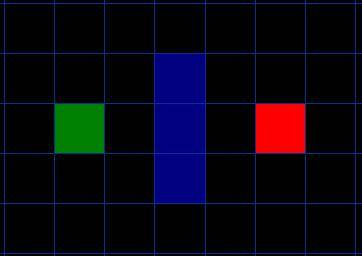（这里的搜索方向有8个方向）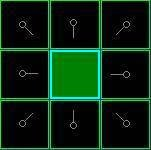G值（耗费值）：指从起点走到该点要耗费的值。

H值（预测值）：指从该点走到终点的预测的值（从该点到终点无视障碍物情况下预测要耗费的值，也可理解成该点到终点的直线距离的值）

（实际上，更复杂的游戏，因为地形不同（例如陷阱，难走的沙地之类的），还会有相应不同的权值：值 = 要走的距离 * 地形权值）

F值（优先级值）：F = G + H

（每个点的左上角为F值，左下角为G值，右下角为H值）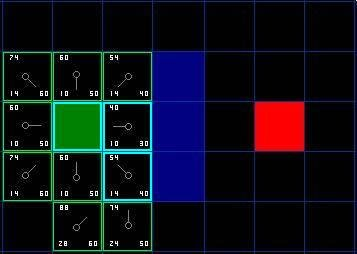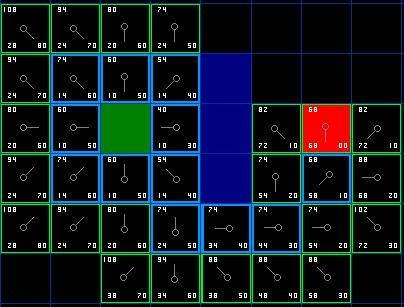（红色标记了便是回溯到的点）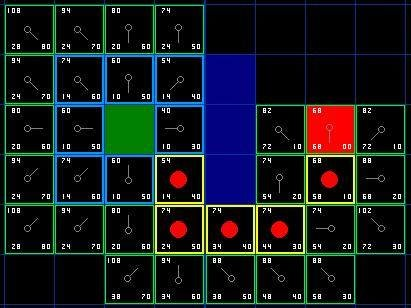# A*算法优化思路

## 障碍物列表，closeList 使用二维表（二维数组）

//假设已经定义Width和Height分别为地图的长和宽
bool barrierList[Width][Height];
bool closetList[Width][Height];

if(barrierList[Xa][Yb]&&closeList[Xa][Yb])来判断。

# A*算法实现（C++代码）

#include <iostream>
#include <list>
#include <vector>
#include <queue>

const int width = 30;            // 地图长度
const int height = 100;          // 地图高度
char mapBuffer[width][height];   // 地图数据
int depth = 0;                   // 记录深度
const int depthLimit = 2000;     // 深度限制

struct OpenPoint {
int x;
int y;
int cost;                 // 耗费值
int pred;                 // 预测值
size_t father;            // 父节点索引值
OpenPoint() = default;
OpenPoint(int pX, int pY, int endX, int endY, int c, size_t fatherIndex) : x(pX), y(pY), cost(c), father(fatherIndex) {
// 相对位移x,y取绝对值
int relativeX = std::abs(endX - pX);
int relativeY = std::abs(endY - pY);
// x,y偏移值n
int n = relativeX - relativeY;
// 预测值pred = (max–n)*14+n*10+c
this->pred = std::max(relativeX, relativeY) * 14 - std::abs(n) * 4 + c;
}
};

// 存储OpenPoint的内存空间
std::vector<OpenPoint> pointPool;
// 创建一个开启点
size_t createOpenPoint(int pX, int pY, int endX, int endY, int c, size_t fatherIndex) {
pointPool.emplace_back(OpenPoint(pX, pY, endX, endY, c, fatherIndex));
return pointPool.size() - 1;
}

// 记录障碍物+关闭点的二维表
bool closeAndBarrierList[width+1][height+1];
// 是否在障碍物或者closelist
bool inBarrierAndCloseList(int pX, int pY) {
if (pX < 0 || pY < 0 || pX >= width || pY >= height)
return true;
return closeAndBarrierList[pX][pY];
}

// 比较器，用以优先队列的指针类型比较
struct OpenPointCompare {
bool operator()(size_t a, size_t b) {
return pointPool[a].pred > pointPool[b].pred;
}
};
// openlist 使用最大优先队列
std::priority_queue<size_t, std::vector<size_t>, OpenPointCompare> openlist;

// 开启检查 openPoint
void open(size_t openPointIndex, int endX, int endY) {
int px = pointPool[openPointIndex].x;
int py = pointPool[openPointIndex].y;
// 如果目标在障碍物或者closelist
if (inBarrierAndCloseList(px, py)) {
return;
}
// 移入closelist
closeAndBarrierList[px][py] = true;

// openPoint的开销
int pcost = pointPool[openPointIndex].cost;
// 八个方向以及方向对应的开销
const int dir = { {1,0},{0,1},{-1,0},{0,-1},{1,1},{ -1,1 },{ -1,-1 },{ 1,-1 } };
const int cost = { 10,10,10,10,14,14,14,14 };
// 检查p点八方的点
for (int i = 0; i < 8; ++i)
{
int toOpenX = px + dir[i];
int toOpenY = py + dir[i];
openlist.push(createOpenPoint(toOpenX, toOpenY, endX, endY, pcost + cost[i], openPointIndex));
}
}

// 开始搜索路径
std::list<size_t> findway(int startX, int startY, int endX, int endY) {
// 创建并开启一个父节点
openlist.push(createOpenPoint(startX, startY, endX, endY, 0, -1));
size_t endIndex= -1;
// 重复寻找预测和花费之和最小节点开启检查
while (!openlist.empty())
{
// 深度+1
depth++;
// 若超出一定深度，则搜索失败
if (depth >= depthLimit) {
return std::list<size_t>();
}

size_t openPointIndex = openlist.top();
// 将父节点从openlist移除
openlist.pop();

// 找到终点后，则停止搜索
if (pointPool[openPointIndex].x == endX && pointPool[openPointIndex].y == endY) {
endIndex = openPointIndex;
break;
}
open(openPointIndex, endX, endY);
}
// 返还一条路径
for (size_t index = endIndex; index != -1; index = pointPool[index].father) {
}
}

// 创建地图
void createMap() {
for (int i = 0; i < width; ++i)
for (int j = 0; j < height; ++j) {
// 五分之一概率生成障碍物，不可走
if (rand() % 5 == 0) {
mapBuffer[i][j] = '*';
closeAndBarrierList[i][j] = true;
}
else {
mapBuffer[i][j] = ' ';
closeAndBarrierList[i][j] = false;
}
}
}

// 打印地图
void printMap() {
for (int i = 0; i < width; ++i) {
for (int j = 0; j < height; ++j)
std::cout << mapBuffer[i][j];
std::cout << std::endl;
}
std::cout << std::endl << std::endl << std::endl;
}

int main() {
// 起点
int beginX = 0;
int beginY = 0;
// 终点
int endX = 29;
int endY = 99;
// 创建地图
createMap();
// 保证起点和终点都不是障碍物
mapBuffer[beginX][beginY] = mapBuffer[endX][endY] = ' ';
closeAndBarrierList[beginX][beginY] = closeAndBarrierList[endX][endY] = false;
// A*搜索得到一条路径
std::list<size_t> road = findway(beginX, beginY, endX, endY);
// 将A*搜索的路径经过的点标记为'O'
for (size_t index : road) { mapBuffer[pointPool[index].x][pointPool[index].y] = 'O'; }
// 打印走过路后的地图
printMap();
system("pause");
return 0;
}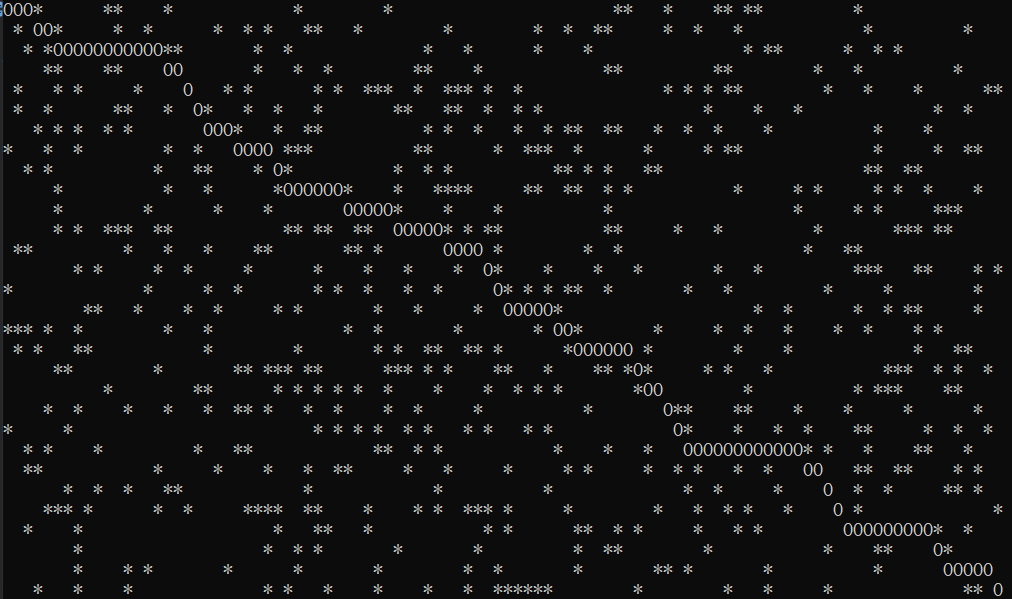# 额外

#### 游戏AI 系列文章：https://www.cnblogs.com/KillerAery/category/1229106.html

posted @ 2018-06-30 08:29  KillerAery  阅读(9304)  评论(4编辑  收藏  举报